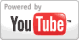Area of a Parallelogram - Geometry Lesson
Grade: 6 - 12 | In this lesson, learn the formula for the area of a parallelogram, and solve problems using this formula. Right triangle formulas and trigonometry are used extensively in the problems in this lesson.Add to My DashboardGeometry### Search Videos & Games